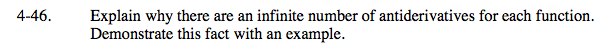### Home > CALC > Chapter 4 > Lesson 4.2.1 > Problem4-46

4-46.

Explain why there are an infinite number of antiderivatives for each function. Demonstrate this fact with an example. Homework Help ✎The +C represents a vertical shift.

Explain why two functions that are the same in every way except for their vertical position would have the exact same slope at every value of x.